Close

• The colors may differ slightly from the original.

Past Model

10 + 3 digits
10-digit mantissa + 3-digit exponential display.

## Features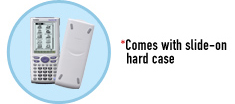### User-friendly Interface

#### Pen Touch Operation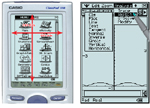Intuitive stylus operation for entry of values and expressions, selection of menu commands, drag-and-drop copying of values and expressions, and much more. A big 160 × 240-dot LCD simplifies operation and shows more data per screen.

#### Natural Textbook Input and Output

Fractions, powers, and square roots, as well as high-level mathematical expressions such as log, Σ, ∫, d/dx, lim, matrices, F (Fourier transforms), and L (Laplace transforms) can be entered and displayed just as they appear in your textbook. An on-screen soft keyboard helps to simplify entry of complex expressions.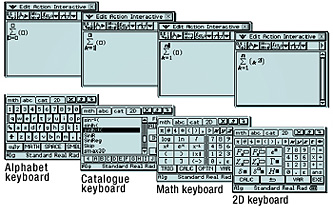#### Advanced CAS (Computer Algebra System)

Base- n capabilities have been added for general-purpose numerical and mathematical calculations. Natural input/output mathematical functions have been expanded to include F (Fourier transforms), L (Laplace transforms), δ, Γ, H, and more.

#### Differential Equation Application

The solution set of a differential equation can be represented graphically as a vector field, and solution curves can be drawn by providing initial conditions for the equation. First, second, and n -th order differential equations are supported.

#### Financial Application

This ClassPad 330 application provides you with a total of 15 different financial calculations, including simple/compound interest, cash flow, amortization, depreciation, bond calculation, operating/financial leverage, and more.

Collected data can be organized and tabulated for analysis after statistical graphing is complete. Spreadsheet data also can be used in table calculations. In addition, ClassPad 330 adds the following functions: search, sort, data import from and export to lists, matrices, and variables, CellIf, and Histogram/Box-whisker graphing.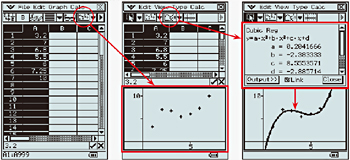### Geometry Application

#### Geometric Graphing

Students can learn the general theorems by drawing figures, and can confirm that a theorem still holds true even when the form of the triangle is altered.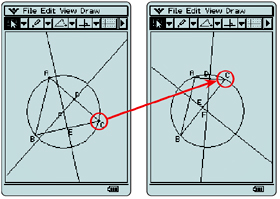#### Animation

An Animation function provides the means to move geometric figures drawn on the screen. You can even plot the locus for a particular point of the animation. The screenshot shows an example where Point D is plotted as the locus for Point E moving on Line AB.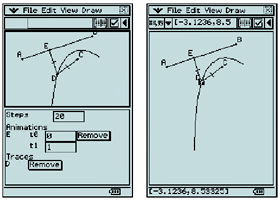#### Geometric Graphing Using Drag & Drop

Dropping a geometric figure into the Main application window will produce the numerical data for the figure. Conversely, dropping numerical data into the Geometry window will produce the applicable figure.#### Enhanced Geometric Functions

ClassPad 330 supports drawing of conics using a focus, as well as graphing of polar equations and parametric equations. Enhanced labeling capabilities let you display attached angles, measurement-based calculation results, and more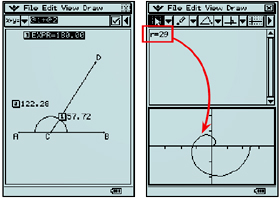### eActivity Application

An eActivity is like a digital worksheet that can be created and worked with on the ClassPad 330. All of the powerful features and capabilities of the ClassPad 330 can be incorporated into an eActivity. In addition to being able to perform the same calculations as the Main application, an eActivity will accept text entry, just like a word processor. Graphs, as well as Geometry and Spreadsheet data also can be stored in an eActivity file.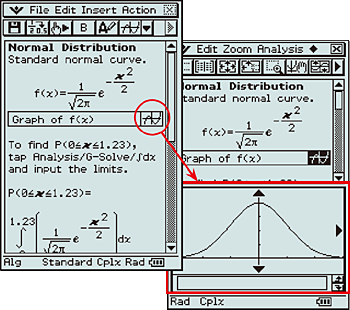### 3D Graph Application

The 3D Graph Application lets you draw rectangular coordinate graphs (z = f (x, y)) and parametric function graphs (xst = f (s, t), yst = f (s, t), zst = f (s, t)). You can split the display screen between a 3D Graph Editor window and 3D Graph window, or enlarge the 3D Graph window to view a larger graph.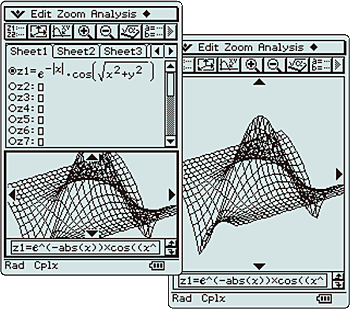## Specifications

### ALGEBRA

• CAS (Computer Algebra System)
• Algebra Assistant
• Fractions
• Transformation (simplify, expand, factor)
• Algebraic (√, x 2 , x –1 , x!, n √, x n )
• Simultaneous equations
• Real and Complex results
• List
• Matrix
• Combination nCr, Permutation nPr
• Exponents (log, ln, 10 x , e x )
• Trigonometrics (sin, cos, tan, sin –1 , cos –1 , tan –1 )
• Function graphing, polar, parametric and x = f (y) equations
• Numeric evaluation of functions in tables
• Graph solve (root, max, intersection, inflection, distance)
• Conics graphs (Parabola, Circle, Ellipse, Hyperbola, General figure)
• Conics graph solve (Focus, Vertex, Directrix, Symmetry, Center, Radius)
• Recursive and explicit sequence numerical tables and plots
• Number Base (base 2 (Bin), 8 (Oct), 10 (Dec) and 16 (Hex))
• Laplace transform, Fourier transform, Fast Fourier transform (FFT)

### CALCULUS

• Hyperbolics (sinh, cosh, tanh, sinh –1 , cosh –1 , tanh –1 )
• Integration, Differential
• Differential equation
• Σ, ∏, lim
• Dirac Delta, Heaviside Unit Step, Gamma

### STATISTICS

• List-based one- and two-variable statistical analysis
• Statistical regression calculations
• Statistical plot (Scatter Plot, xyLine, Normal Probability Plot, Histogram, Box-whisker plot)
• Statistical regression graphs
• Advanced statistical calculations (Tests, Confidence Intervals and Distribution calculations)

### GEOMETRY

• Constraint geometry (for education)
• Construction figures (Perpendicular, Midpoint, Intersection, Angle Bisector, Parallel, Tangent to Curve)
• Geometry figures (Circle, Arc, Ellipse, Hyperbola, Parabola, Triangle, Rectangle, n -gon, Point, Line Segment, Ray, Vector)
• Geometry animation
• Numeric evaluation of geometry animation in tables
• Labels (Text, Attached Angle, Measurement, Expression)

### eACTIVITY APPLICATION

• eActivity creation
• eActivity exploration (execution)

### OTHER USEFUL FEATURES

• Drag & drop
• Natural format input of equations and expressions
• Natural format display of results
• Math, Alphabet, 2D soft keyboards
• Command catalog soft keyboard
• Calculation History
• Mantissa + exponent: 15 + 3
• Interactive manipulation for solving equations
• 3-dimensional graphs
• Differential equation graphs
• Numeric equation solver
• Financial calculations
• Presentation feature
• Program storage capacity: 500KB (max)
• Full screen display/Split screen display
• User-defined variable
• User-defined function (extends built-in functions)
• Folder-based memory management
• Unit-to-unit screen image transfer
• Resetting/Initializing memory
• Selectable display language
• Auto Power Off (APO)
• Ending Screen/User-defined Ending Screen
• Bundled program-link software FA-CP1: This data transfer software runs on a Windows computer. You can use it to transfer certain ClassPad unit files and to back up all ClassPad unit data on your computer. You can also transfer ClassPad unit screen captures to your computer.

### HARDWARE

• Dimensions: 21.0 (H) × 84.0 (W) × 189.5 (D) mm
• Approximate weight: 280g
• Battery type: Four AAA-size batteries LR03 (AM4)
• Battery life: Approx. 140 hours continuous operation (assuming 5 minutes calculation and 55 minutes display per hour)
• Display type: 160 × 240-dot LCD
• Touch Panel (Pen Touch Operation)
• User-available RAM: 500KB
• User-available Flash ROM (Add-in area): 5.3MB
• Data communication (via USB and 3-pin cables)
• USB cable for connecting with PC
• 3-pin cable for connecting with other ClassPad unit or EA-200

### OPTIONS

• ClassPad Manager Version 3.0 FA-CP300A/B
• EA-200 Data Analyzer

## Specifications

•Natural textbook display Display expression same as textbook.

•10 + 3 digits 10-digit mantissa + 3-digit exponential display.

•Dot matrix display High-resolution screen provides beautiful looking graphs every time.

•Icon menu Specify the operation you want to perform by selecting an icon or inputting a number.

•Multi-replay Quick and easy recall of previously executed formulas for editing and re-execution.

•Plastic keys Designed and engineered for easy operation.

•Data communication with a personal computer Allows data communication with a personal computer.

•List based STAT-data editor Viewing and editing of input data in list format, showing data groups (x-data, y-data, frequency) and surrounding data.